# Cylindrical Capacitor

## Capacitance of a Cylindrical Power Cable

Electrical Power Cable is a very popular example of cylindrical capacitor. In a power cable, there is a conductor at the center surrounding which there is insulating layer. Outer surface of the cable is generally covered with metallic cover which is grounded.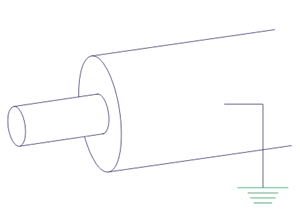Let us also consider, at any instant due to current in the conductor, the charge of the cable is Q coulomb per meter. The radius of conductor and outer radius of cable are r1 and r2 respectively.

Now for calculating capacitance of this cylindrical capacitor, consider concentric imaginary cylinder of radius x meter. Where,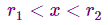Now, the surface area of 1m long such imaginary cylinder is,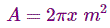Now as per definition, the flux density on that surface will be,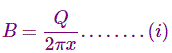Again, as per definition, electrical field intensity at any point on that imaginary surface will be,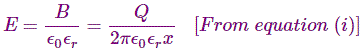Again, electrical field intensity is defined as the ratio incremental change in voltage to incremental change in distance.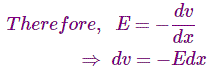Now integrating both sides from r1 to r2 we get,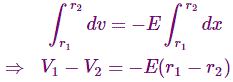Where, surface voltage of conductor of radius r1 m is V1 volt and surface voltage of outer surface of cable of radius r2 m is V2 volt.
Now, if outer surface is grounded, then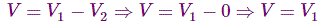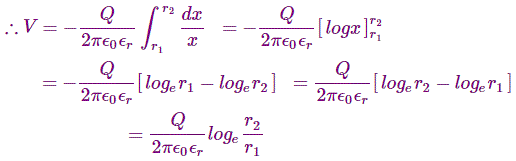Now, capacitance of the cable per unit length i.e. per meter is given as,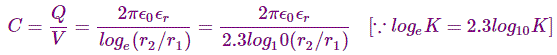This is expression of capacitance per unit length i.e. per meter of the cable.
Hence, the capacitance of L meter cable will be,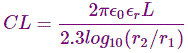Want To Learn Faster? 🎓
Get electrical articles delivered to your inbox every week.
No credit card required—it’s 100% free.